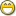1. Wrap-around search algorithm

This proved far harder than I ever imagined possible, any improvements welcome.

The following is a simplified testbed.
First simplification: text is "0000".."1111" and "find" is whether or not text[1..4] is '1'.
Second simplification: we set "start" explicitly in the testing loop, maybe unlike real use.
(Technically we shouldn't need to reset wrappable in this testbed, but abs. rqd. in real use)
Starting from 1..4, with N=sum of 1s, we want f3find() to get N/eof/N/eof, iyswim.
The challenge is(was) to get all 64 test cases to work, then repeat searching backwards.

Anyway, a second set of eyes, before I try applying this to Edix/Edita, anything that simplifies it or makes it any easier to understand, or OE-compatible, thankx.

sequence text
integer start, current, wrappable = 1

function f3find(integer direction)
--
-- direction should be +/-1. [existing code uses 0 to mean "from line 1"]
-- return "next/prev" '1' in text, wrapping around relative to start.
-- return -1 at "eof", aka "start", allowing restart.
--
integer limit = iff(direction<0?1:length(text)),
begin = iff(direction>0?1:length(text))

current += direction
bool high = wrappable and compare(start,current)!=direction
limit = iff(high ? limit : start-(wrappable=0)*direction)
for i=current to limit by direction do
if text[i]='1' then current=i return i end if
end for
if high then
wrappable = 0
limit = start-direction
for i=begin to limit by direction do
if text[i]='1' then current=i return i end if
end for
end if
current = start-direction
wrappable = 1
return -1
end function

constant TRIES=4
integer fails = 0, total = 0
for i=0 to 15 do
text = sprintf("%04b",i)
integer N = sum(sq_eq(text,'1'))
?{text,N}
for direction=+1 to -1 by -2 do
for j=1 to 4 do
start = j
current = j-direction
wrappable = 1
sequence s = {}
for t=1 to (N+1)*TRIES do
s &= f3find(direction)
end for
total += 1
if s[\$]!=-1 or sum(sq_eq(s,-1))!=TRIES then
s &= {"9/0"}
fails += 1
?{"s=",s}
end if
--          ?s
end for
end for
end for
printf(1,"fails: %d, pass: %d/%d\n",{fails,total-fails,total})

output:

{"0000",0}
{"0001",1}
{"0010",1}
{"0011",2}
{"0100",1}
{"0101",2}
{"0110",2}
{"0111",3}
{"1000",1}
{"1001",2}
{"1010",2}
{"1011",3}
{"1100",2}
{"1101",3}
{"1110",3}
{"1111",4}
fails: 0, pass: 128/128

2. Re: Wrap-around search algorithm

some oE vs Phix syntax differences

• oE does not have %b format code
• oE does not have iff) function
• oE does not have bool
• oE does not have negative index values as in: s[\$]!=-1

I can't figure out what you are doing!

_tom

3. Re: Wrap-around search algorithm

Pseudo code:

This way you can even highlight all the matches, but make the current one a bright highlight:

sequence matches
sequence lastStrFind = ""

procedure find(sequence strFind = lastStrFind, integer bDirection)
integer cursorPos = current_cursor_position()

if length(strFind) = 0 then
if length(lastStrFind) = 0 then
return
else
strFind = lastStrFind
end if
end if

matches = match_all( strFind, docText )

if bDirection = Forward then
if cursorPos < matches then
-- at the top, just go to first found

elsif cursorPos > matches[\$] then
-- at end of document, wrap to first in matches[]

else
for t=1 to length(matches) docText
if matches[t] > cursorPos then
highlightFound( matches[t-1], length(strFind) )
exit
end if
end for
end if
else
-- do it backwards style
end if

lastStrFind = strFind
end procedure

This way you don't have to keep the matching string in memory, but it doesn't allow you to highlight all the matches:

pos = match( strFind, docText, currentCursorPosition )

if pos = 0 then
pos = match( strFind, docText, 0 )
if pos = 0 then
end if
else
setHighlight( pos, length(strFind) )
end if

I also like in MS VS Code how if I select text, it lightly highlights all matching text in the document. If I highlight a variable name, for example, it subtly shows me all places on the page where that variable appears. Very nice!

Looks like Notepad++ also does the selection highlighting.